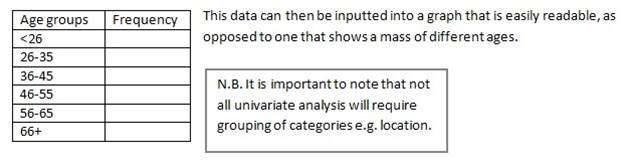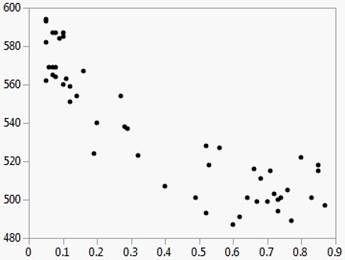# Differentiate between Univariate, Bivariate and Multivariate

Univariate, bivariate and multivariate are the various types of data that are based on the number of variables. Variables mean the number of objects that are under consideration as a sample in an experiment. Usually there are three types of data sets. These are;

Univariate Data Analysis:

Univariate data is used for the simplest form of analysis. It is the type of data in which analysis are made only based on one variable. For example, there are sixty students in class VII. If the variable marks obtained in math were the subject, then in that case analysis will be based on the number of subjects fall into defined categories of marks.

Bivariate Data Analysis:

Bivariate data is used for little complex analysis than as compared with univariate data. Bivariate data is the data in which analysis are based on two variables per observation simultaneously.

Multivariate Data Analysis:

Multivariate data is the data in which analysis are based on more than two variables per observation. Usually multivariate data is used for explanatory purposes.

Applications

Univariate analysis is a form of quantitative, statistical, evaluation. This method of analysis separately studies the findings regarding each variable in a data set, and therefore each individual variable is summarised on its own.

Consequently univariate data does not look at relationships between various variables (like bivariate and multivariate analysis); its sole purpose is to describe one aspect of a piece of research. The easiest way of consolidating the data for one variable is either in a frequency distribution table or bar graph, although other formats can be used (e.g. pie chart, histogram etc.). This means that the number of cases in a particular category (variable) are analysed in one of these chosen means of presentation.

Using the question ‘what’s the age of individuals in the village?’ you may get a wide range of numbers, so the best thing to do is to group the ages (once you have decided on the categories to use) and tally them in a frequency table.After gathering the data for the particular variable in question a researcher can then determine a number of measures regarding the distribution of the data, including: the median, mean, standard deviation, and the minimum and maximum values. Having these values allows a researcher to carry out a number of tests in order to establish a clearer picture of the distribution of the data

BIVARIATE ANALYSIS

It is one of the simplest forms of quantitative (statistical) analysis. It involves the analysis of two variables (often denoted as X, Y), for the purpose of determining the empirical relationship between them.

Bivariate analysis can be helpful in testing simple hypotheses of association, however, by itself, it cannot determine causality. Bivariate analysis can help determine to what extent it becomes easier to know and predict a value for one variable (possibly a dependent variable) if we know the value of the other variable (possibly the independent variable) (see also correlation and simple linear regression).

Bivariate analysis can be contrasted with univariate analysis in which only one variable is analysed.Like univariate analysis, bivariate analysis can be descriptive or inferential. It is the analysis of the relationship between the two variables.Bivariate analysis is a simple (two variable) special case of multivariate analysis (where multiple relations between multiple variables are examined simultaneously).

The Bivariate platform shows the relationship between two continuous variables. It is the continuous by continuous personality of the Fit Y by X platform. The word bivariate simply means involving two variables instead of one (univariate) or many (multivariate).

The Bivariate analysis results appear in a scatterplot. Each point on the plot represents the X and Y scores for a single subject; in other words, each point represents two variables. Using the scatterplot, you can see at a glance the degree and pattern of the relationship between the two variables. You can interactively add other types of fits, such as simple linear regression, polynomial regression, and so on. Example of Bivariate AnalysisMULTIVARIATE

Multivariate statistical analysis refers to multiple advanced techniques for examining relationships among multiple variables at the same time. Researchers use multivariate procedures in studies that involve more than one dependent variable (also known as the outcome or phenomenon of interest), more than one independent variable (also known as a predictor) or both. Upper-level undergraduate courses and graduate courses in statistics teach multivariate statistical analysis. This type of analysis is desirable because researchers often hypothesize that a given outcome of interest is effected or influenced by more than one thing.

# Types

There are many statistical techniques for conducting multivariate analysis, and the most appropriate technique for a given study varies with the type of study and the key research questions. Four of the most common multivariate techniques are multiple regression analysis, factor analysis, path analysis and multiple analysis of variance, or MANOVA.

# Multiple Regression

Multiple regression analysis, often referred to simply as regression analysis, examines the effects of multiple independent variables (predictors) on the value of a dependent variable, or outcome. Regression calculates a coefficient for each independent variable, as well as its statistical significance, to estimate the effect of each predictor on the dependent variable, with other predictors held constant. Researchers in economics and other social sciences often use regression analysis to study social and economic phenomena. An example of a regression study is to examine the effect of education, experience, gender, and ethnicity on income.

# Factor Analysis

Factor analysis is a data reduction technique in which a researcher reduces a large number of variables to a smaller, more manageable, number of factors. Factor analysis uncovers patterns among variables and then clusters highly interrelated variables into factors. Factor analysis has many applications, but a common use is in survey research, where researchers use the technique to see if lengthy series of questions can be grouped into shorter sets.

# Path Analysis

This is a graphical form of multivariate statistical analysis in which graphs known as path diagrams depict the correlations among variables, as well as the directions of those correlations and the "paths" along which these relationships travel. Statistical software programs calculate path coefficients, the values of which estimate the strength of relationships among the variables in a researcher's hypothesized model.

# MANOVA

Multiple Analysis of Variance, or MANOVA, is an advanced form of the more basic analysis of variance, or ANOVA. MANOVA extends the technique to studies with two or more related dependent variables while controlling for the correlations among them. An example of a study for which MANOVA would be an appropriate technique is a study of health among three groups of teens: those who exercise regularly, those who exercise on occasion, and those who never exercise. A MANOVA for this study would allow multiple health-related outcome measures such as weight, heart rate, and respiratory rates.

# Benefits

Multivariate statistical analysis is especially important in social science research because researchers in these fields are often unable to use randomized laboratory experiments that their counterparts in medicine and natural sciences often use. Instead, many social scientists must rely on quasi-experimental designs in which the experimental and control groups may have initial differences that could affect or bias the outcome of the study. Multivariate techniques try to statistically account for these differences and adjust outcome measures to control for the portion that can be attributed to the differences.

# Considerations

Statistical software programs such as SAS, Stata, and SPSS can perform multivariate statistical analyses. These programs are frequently used by university researchers and other research professionals. Spreadsheet programs can perform some multivariate analyses, but are intended for more general use and may have limited abilities than a specialized statistical software package.